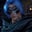Related Tags

typescript
communitycreator

# What are tuples in TypeScript?Gutha Vamsi Krishna

A tuple is another sort of array that knows how many elements will be present in the tuple. Tuples also know what data types are present and which position they are at.

### Syntax

let tulpleName:[data type, data type] = [value,value]


### Code

The code snippet below demonstrates how to create a tuple and assign values to it.

• datesAndMonths: the name of the tuple.
• [number,string]: the data types that will be stored in the tuple in the given order.
• [5,"january"]: the values assigned to the tuple.

Note that we provide 5 first since it is a number, and then january, as it is a string. We follow the order in which we provide data types.

//tuple creation
let datesAndMonths:[number,string] = [5,"january"]
console.log(datesAndMonths)

Let’s try changing the order of the values. In the following code snippet, we access past a valid index and get an error.

//changing the order of the elements.
let datesAndMonths:[number,string] = ["january",5]
console.log(datesAndMonths)

### Indexing

• Every element in a tuple is marked with some index.

• Indexing starts with 0 and ends with n-1, where n is the number of elements in the tuple.

• Indices are assigned from left to right.

• If we have a tuple of elements [2,3,5,7], then:

• element 2 is indexed as 0

• element 3 is indexed as 1

• element 5 is indexed as 2

• element 7 is indexed as 3

### How to access elements

We use indexes to access the elements inside a tuple.

let primes:[number,number,number] = [2,3,5]

//accessing first element in tuple
console.log(primes)

//accessing second element in tuple
console.log(primes)

In the following code snippet, we access past a valid index and get an error.

let primes:[number,number,number] = [2,3,5]

//access element, past the number of elements present
// in tuple will throw error
console.log(primes)

### How to destructure tuples

We can de-structure tuples in TypeScript as we do in JavaScript. This is useful when we want to assign tuples to individual variables, or if we don’t want to use the entire data set that we are getting.

In the following code snippet:

• data is the name of the tuple.

• [number,string,string,number] are the types that the tuple holds in the same order.

• [5,"abc","def",6] are the values assigned to the tuple.

• Line 2 destructures the tuple data.

• Line 4 prints the stringFromData variable destructured from data.

let data:[number,string,string,number] = [5,"abc","def",6]
let [numberFromData, stringFromData] = data;

console.log(stringFromData)

### Rest elements

Sometimes, one specific data type element may occur many times in a tuple. In that case, it would be tedious to specify that data type to the tuple. Here, we can use rest elements to make things easier.

In the following code snippet, we create a custom tuple type StringBooleans from [string, ...boolean[]] and assign that type to variable a.

We can pass as many Booleans as we want, so we can assign the first value as a string and the remaining elements as Booleans.

type StringBooleans = [string, ...boolean[]];

let a:StringBooleans = ["abc",true,false,true,false,true]

console.log(a)

We can restrict the tuple to read-only so that when we pass the tuple anywhere, nobody can modify the original tuple.

In the following code snippet, we make data read-only with the readonly keyword. In line 5, we try to modify the read-only tuple data. This will raise an error.

Please try the following code in TypeScript Playground.

//making tuple as read only

//trying to modify tuple
data = "qwerty";

RELATED TAGS

typescript
communitycreator

CONTRIBUTORGutha Vamsi Krishna
RELATED COURSES

View all Courses

Keep Exploring

Learn in-demand tech skills in half the time